# The CVX returns NAN for Least square regression

I’m solving a least-squares regression problem with equality constraints. the CVX returns NAN for all values; however, when I solved it with a closed-form solution approach, there is a solution to this problem
The code is as follows:
x::(531)
LHS_training:: (9000
53)
Library_constraint:: (9000*53)

``````cvx_begin quiet

variable x(n);
minimize( norm(LHS_training-Library_training*x) );
subject to

Library_constraint*x == LHS_constraint;

if k>1
x(smallinds)==0;
end
cvx_end;
``````

The first piece of advice is to not use the `quiet` option. That way you can see the CVX and solver output. Perhaps the problem was reported as infeasible? if so, that should be in the output when you remove `quiet`.

If the problem is reported as infeasible, you can follow the advice at Debugging infeasible models - YALMIP, all of which except for section 1 also applies to CVX. In particular, try solving the problem without the objective. Do you expect those constraints to be satisfied exactly, as opposed to in some kind of least squares or other approximate sense? Because that is what you are requiring in your CVX code. You haven’t told us how you calculated the closed-form solution - are you sure those constraints are actually satisfied with your supposed solution?

Hopefully you are following the advice given to you previously to use CVX 2.2 rather than CVX 3.0beta, which due to bugs is known to not always handle constraints correctly.

thanks,
the output is infeasible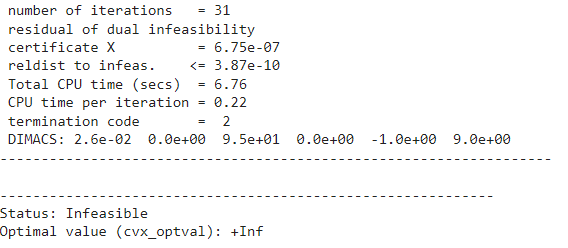I tried to solve it without objective function and the output is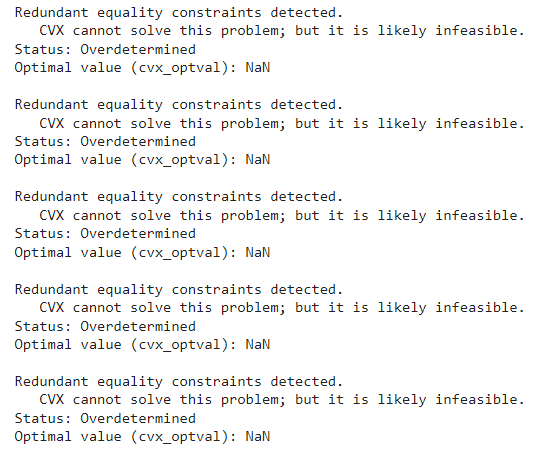When I tried to reduce number of constraints to only 10, it worked but the results are not good

This problem represents a dynamic system, and I was able to find the x with the Euler-Lagrange algorithm.

Also, the Two matrices (Training and Constraint are ill conditioned)

Please, @Mark_L_Stone, can you tell me how can I add some tolerance on achieving the constraints.

You could change the equality constraint to

`norm(Library_constraint*x - LHS_constraint) <= upper_limit`

where `upper_limit` is a value of your choosing. You could solve this “parametrically” for several different values of `upper_limit`. You can also chose which norm to use (two-norm, as I show, inf, or 1, or any “p” >= 1.

Alternatively, you could add `multiplier*norm(Library_constraint*x - LHS_constraint) ` to the objective function as a penalty term. You can do this parametriically for multiple values of `multiplier` and choose which “p” to use in the norm,

Have you evaluated `norm(Library_constraint*x - LHS_constraint)` for your closed-form solution? Does it also satisfy

``````if k>1
x(smallinds)==0;
end
``````

`
It would not be a bad idea to improve the conditioning of the input data, if you can.

1 Like

Thank you so much,
I evaluated the norm on the closed-form solution, and it satisfied.

I will try to improve the conditioning of the regressor matrix beside applying tolerance to the constraints

@Mark_L_Stone
Error using [cvx/norm]
I’m trying to solve for this objective
minimize( (1/2)* norm( (LHS_training-Library_training*x).^2 ) );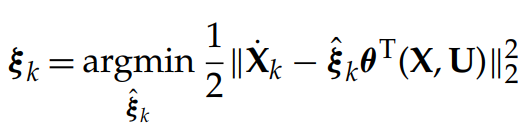but an error appears: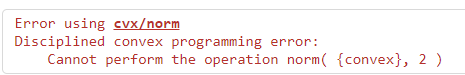Based on the error message, the argument of `norm` is convex, not affine as CVX requires. You haven’t shown the complete code, so i don’t know what the offending item is, nor do I know whether your problem is actually convex and can be formulated in accordance with CVX’s DCP rules. Presuming `x` is an optimization (CVX) variable, it must be the case, given the error message, that one or both of `LHS_training` and `Library_training` are not input data, and were either declared as CVX variables, or are CVX expressions in terms of CVX variables. Perhaps your code has an erroneous variable declaration?

If you succeed in getting the argument of `norm `to be affine, you need to use `square_pos` rather than `^2` to square the norm. But because it is the only term in the objective function, you can just not square it, and you will get the same argmin, and the problem will also be more numerically favorable for the solver (i.e.,more reliably solved).

help cvx/norm

``````Disciplined convex programming information:
norm is convex, except when P<1, so an error will result if
these non-convex "norms" are used within CVX expressions. norm
is nonmonotonic, so its input must be affine.
``````

This is the whole code

for k=1:5 % 5 is the number of iterations
% Run the optimization

``````%cvx_precision low

cvx_begin quiet

variable x(n);
%minimize( norm(LHS_training-Library_training*x) );

minimize( (1/2)* norm(  (LHS_training-Library_training*x).^2  ) );
subject to
%x(1)==0;

%LHS_constraint-1 <= Library_constraint*x <= LHS_constraint+1;

norm(LHS_constraint-Library_constraint*x)<=2.4

x(TF5)==values_TF5;

if k>1
x(smallinds)==0;
end
cvx_end;
% Use thresholding

X=full(x);
smallinds = (abs(X)<lambda);
``````

end

You have not provided a complete reproducible code which generates the error message. I don’t see how this code would produce the error message you showed.

I suggest you follow the advice, provided earlier in this topic, to not use the `quiet` option.

I’m sorry.I removed quiet, but this message appears only,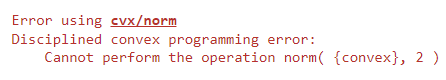`quiet` only matters if CVX calls the solver. Given that error message, CVX never called the solver. But if it ever does, you should look at the solver and CVX output. If you are calling CVX in a loop, and the failure occurs on some iteration after the first, you should be looking at the results, if they become input pt the next iteration.

Thank you very much for your effort

This problem appears at the first iteration using initial matrix of LHS_training & Library_training

You should provide a compete program, with all input data, starting from the beginning of a MATLAB session, if you expect to receive additional help on this. Otherwise, the forum readers have no idea what’s going on.

@Mark_L_Stone

lam=Lambda_5;

[~,n]=size(Library_training);

for i=1:length(lam)

fprintf(‘Lambda %d/%d\n’, i,length(lam))

lambda=lam(i);

for k=1:5

``````cvx_begin quiet

variable x(n);

minimize( (1/2)* norm(  square_pos(LHS_training-Library_training*x) ) );
subject to

norm(LHS_constraint-Library_constraint*x)<=2.4

x(TF5)==values_TF5;

if k>1
x(smallinds)==0;
end
cvx_end;
% Use thresholding

X=full(x);
smallinds = (abs(X)<lambda);
``````

end

if isnan(sum(x))==1
break;
end

SC(:,i)=x;

end

This is the complete Code and I attached the inputs

You FINALLY showed the code which generated the error message. The error message is due to your having `square_pos` in the wrong place, which even if it were allowed, would produce the wrong answer (as for instance, if applied to double precision data)… `square_pos` should be outside of `norm`, not inside it. But as I stated previously, you would be better off not using `square_pos` at all, i.e., don’t square the norm.